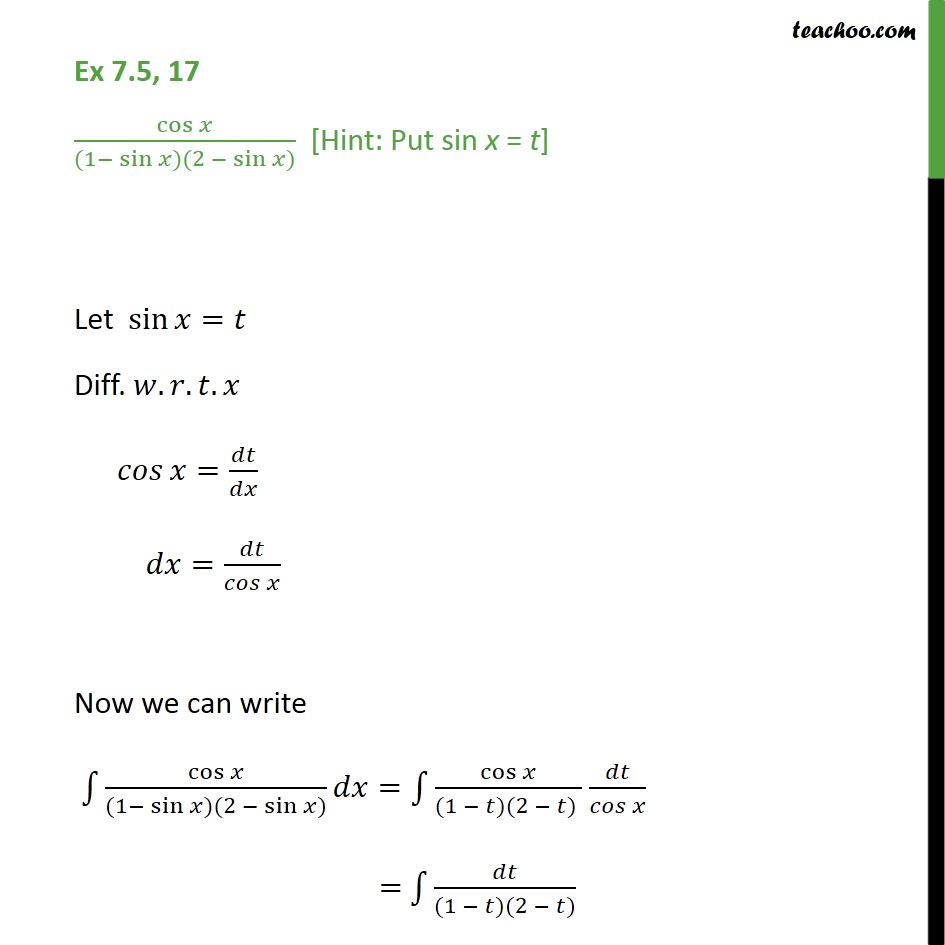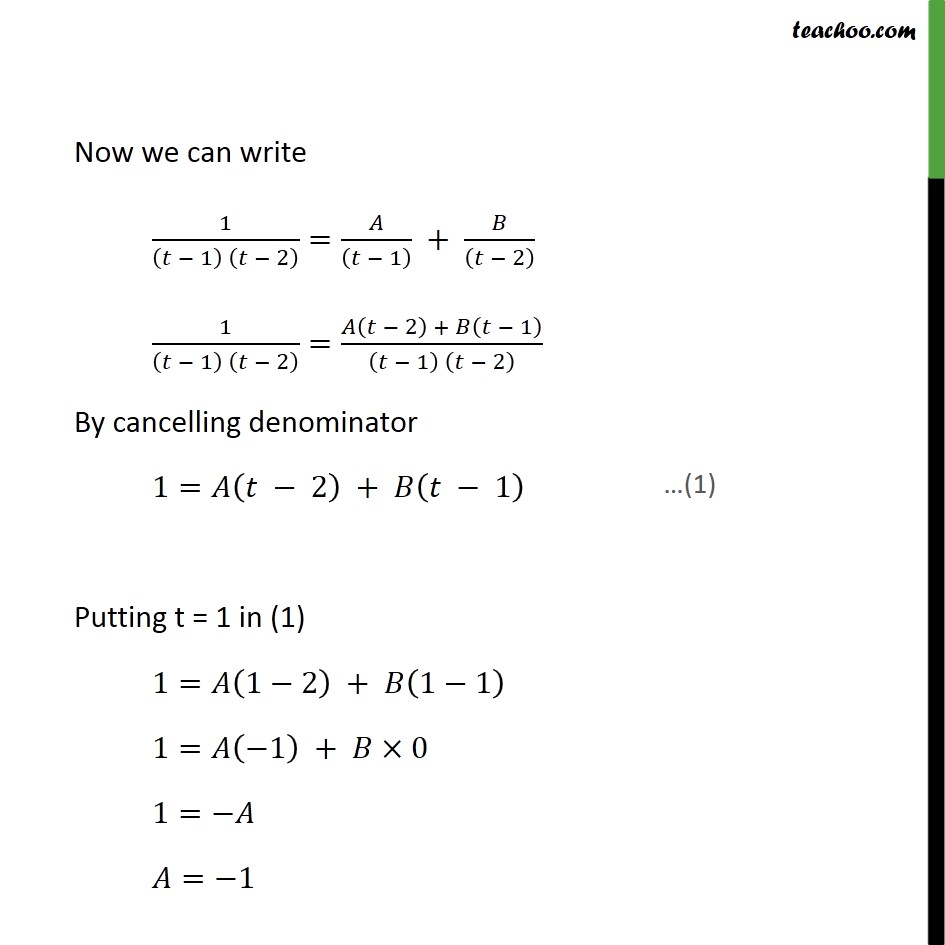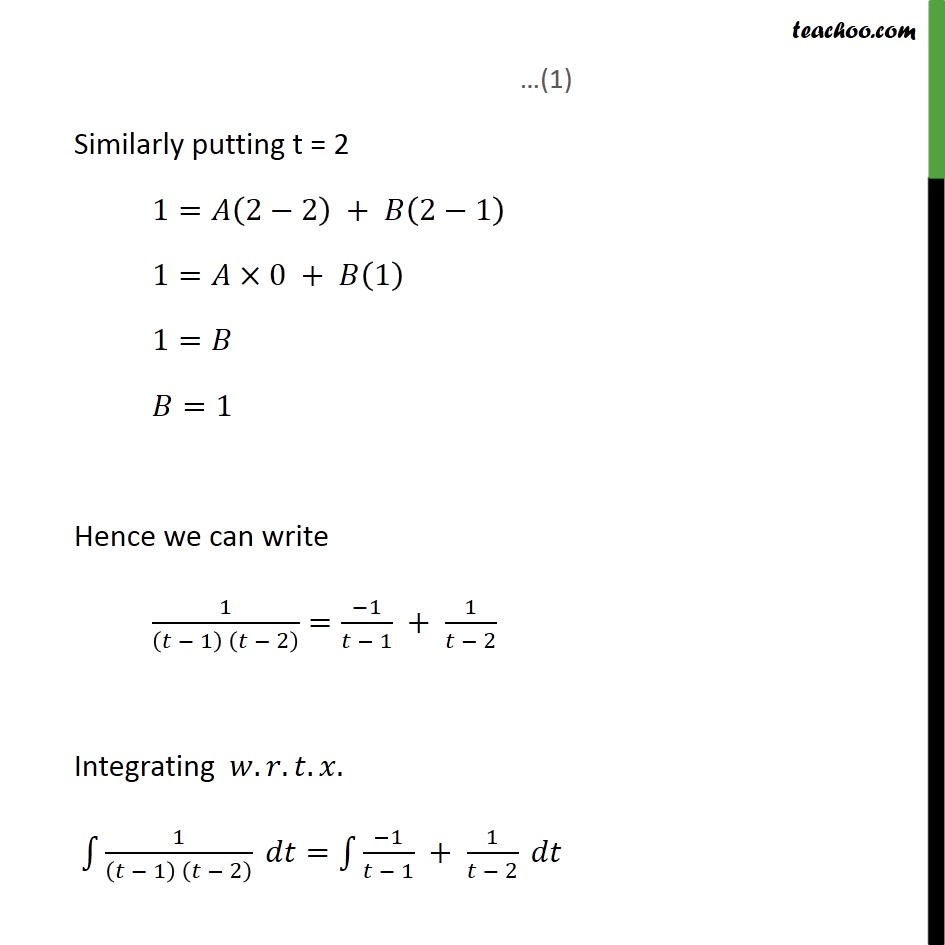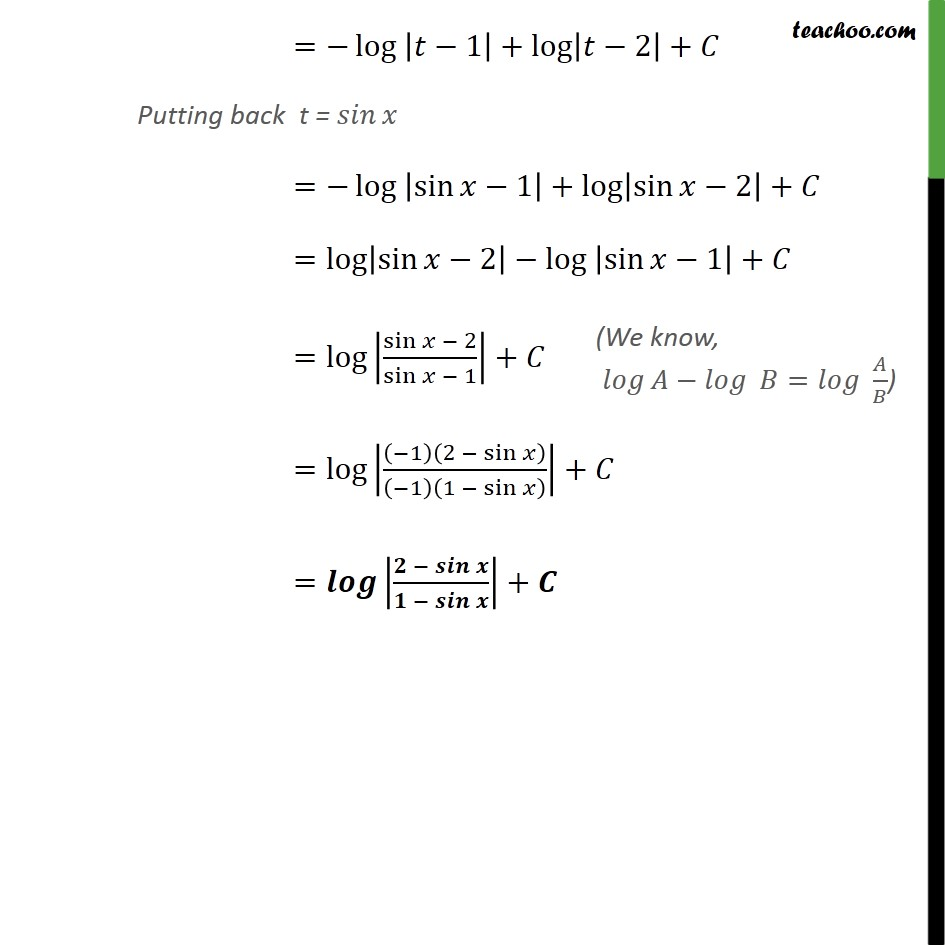Integration by partial fraction - Type 1

Chapter 7 Class 12 Integrals
Concept wiseIntroducing your new favourite teacher - Teachoo Black, at only ₹83 per month

### Transcript

Ex 7.5, 17 cos (1 sin )(2 sin ) [Hint: Put sin x = t] Let sin = Diff. . . . = = Now we can write cos (1 sin )(2 sin ) = cos (1 )(2 ) = (1 )(2 ) Now we can write 1 1 2 = 1 + 2 1 1 2 = 2 + 1 1 2 By cancelling denominator 1= 2 + 1 Putting t = 1 in (1) 1= 1 2 + 1 1 1= 1 + 0 1= = 1 Similarly putting t = 2 1= 2 2 + 2 1 1= 0 + 1 1= =1 Hence we can write 1 1 2 = 1 1 + 1 2 Integrating . . . . 1 1 2 = 1 1 + 1 2 = log 1 + log 2 + Putting back t = = log sin 1 + log sin 2 + = log sin 2 log sin 1 + = log sin 2 sin 1 + = log 1 2 sin 1 1 sin + = +